# Insertion in Linear Array (Beginning, Given Location or End)

Insertion in linear array involves three cases

## Insertion in Linear Array-an element at the beginning of the array

In this case we have to move all the elements one position backwards to make  a hole at the beginning of array. Though the insertion process is not difficult but freeing the first location for new element involves movement of all the existing elements. This is the worst case scenario in insertion in a linear array.

In the below example, array elements from index 1 to index 8 have to moved one position backwards so that the new element 44 can be stored at index 1

###After movement of elements backwards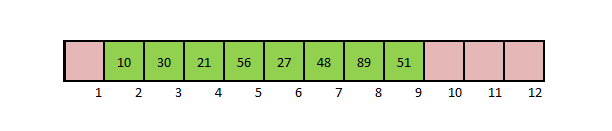### After insertion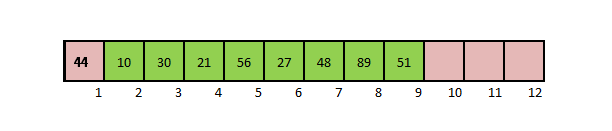## Insertion in Linear Array-  at the end of the array

In this case we don’t have to move any elements since the action here will be just to append the element at the location after the last element.  This is the best case scenario.

In the example array no elements are moved. The new element is stored in index 9.

### Initially### After Insertion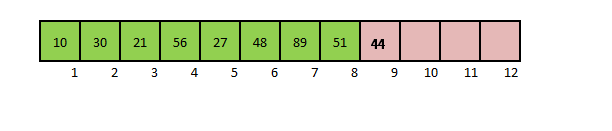## Insertion in Linear Array- at the give position J

Let J be any location in the array ofone element already existing. We have to add  the new element at J position. To do this, starting from J, every element is moved one place backwards so that a hole is created at J and new element can be inserted here. This is the average case scenario.

In the example array ,elements from index J (4) to index 8 have to moved one position backwards so that a new element can be stored at index J(4)

### Initially### After movement of elements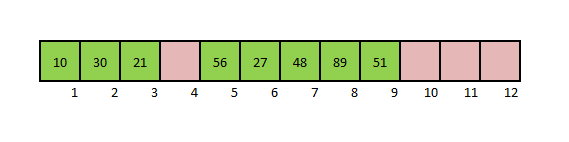### After Insertion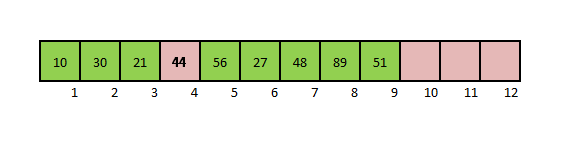Note:- for each one of these three cases the count of elements increases by 1

## Algorithm

```Algorithm InsertLA (DATA, N, ITEM, LOC)
Desc: This algorithm inserts new element ITEM in linear array DATA with N elements
If LOC=1 it means the element has to insert in beginning
If LOC =N+1 it means the element have to be inserted at the end
If LOC = J it means the elements have to be inserted at Jth Location

Begin
Step 1: [Initialize counter I with index of last element]
I=N
Step 2: While I>=LOC repeat steps 3 and 4
Step 3: [Move the current element one position backwards]
DATA[I+1]=DATA[I]
Step 4: [Decrement counter I]
I=I-1
Step 5:[Insert new element at the Location]
DATA[LOC]=ITEM
Step 6:[ Update total under of array elements]
N=N+1
Exit```